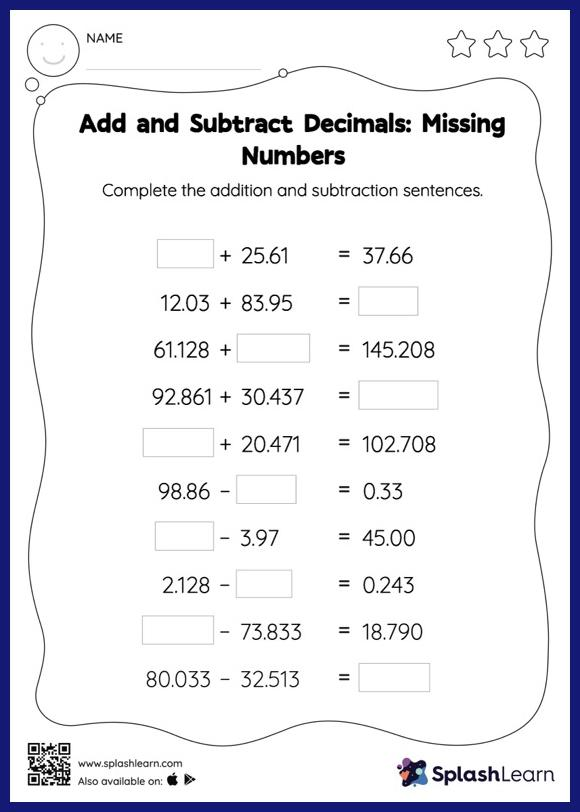# Add and Subtract Decimals: Missing Numbers Worksheet

Home > Add and Subtract Decimals: Missing NumbersChildren use their math skills to complete this add and subtract decimals worksheet. When adding or subtracting decimals on this worksheet, students align the decimal points and use zero as a placeholder. Then to find the missing number in the add and subtract decimals worksheet, students then apply the relationship between addition and subtraction. In each problem, the numbers are laid out in the horizontal format. Students should try to use different strategies involving composing and decomposing numbers to solve these problems. This will help them develop flexibility and fluency.